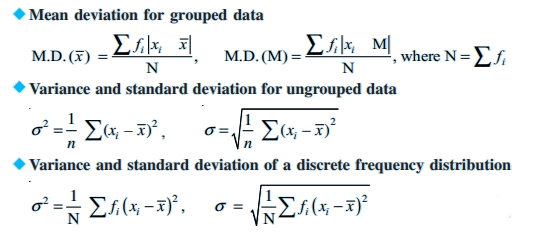# Statistics Class 11 Notes - Chapter 15

## What is the Limit of the Class?

In each class, the starting and end values are called Lower and Upper limit.

### Class Interval

The difference between the lower boundary of a class and the upper boundary of the same class is called the size of the class or class interval.

### Primary and Secondary Data

The data collected by the information collector is known as the primary data, while the data collected by any other guy apart from the collector is known as the secondary data.

### Variable or Variate

A characteristics that vary in magnitude from one specimen to specimen. E.g., strength, physic etc., are variables.

### Frequency

The number of time an observation occurring in the data provided to us is called the frequency of the observation.

### Discrete Frequency Distribution

A frequency distribution is called a discrete frequency distribution if the data are represented in a way where the same measurements of the units are clearly made visible.

### Continuous Frequency Distribution

A frequency in which data are arranged in classes groups which are not exactly measurable.

Range =Maximum -Minimum Value### Important Questions

1. 8 observations have the mean and variance are 10 and 10.25, respectively. If 6 of the observations are 6, 7, 10, 12, 12 and 13, find the remaining 2 observations.
2. 8 and 16 are the mean and variance of 7 observations respectively. Find the remaining two observations, if five of the observations are 2, 4, 10, 12, 14
3. 8 and 4 are the mean and standard deviation of 6 observations respectively. If 3 is multiplied to each observation, find the new mean and new standard deviation of the resulting observations.
4. Find the mean deviation about the mean for the data below:
• 5,3, 9, 11, 12, 14 18, 20,42
• 24, 30, 90, 73, 99, 54, 32, 48,61

## Frequently asked Questions on CBSE Class 11 Maths Notes Chapter 15: Statistics

### What is a ‘Frequency polygon’?

A frequency polygon is a line graph of class frequency plotted against class mid-point.

### What is a ‘Median’?

The median is the middle number in a sorted, ascending or descending, list of numbers.

### What are some of the uses of ‘Statistics’?

Statistics is used in the tudy and manipulation of data, including ways to gather, review, analyse and draw conclusions from data.